# Minimize the short-circuit current pulse in a hot-swap controller

## Maxim MAX4272

,

Because of internal circuit-breaker delay and limited MOS-gate pulldown current, many hot-swap controllers do not limit current during the first 10 to 50 µsec following a shorted output. The result can be a brief flow of several hundred amperes. A simple external circuit can counter this problem by minimizing the initial current spike and terminating the short circuit within 200 to 500 nsec. A typical 12 V, 6 A, hot-swap-controller circuit contains, as do many others, slow and fast comparators with trip thresholds of 50 and 200 mV (Figure 1). The 6-mΩ sense resistor, RS, allows a nominal slow-comparator trip at 8.3 A for overload conditions and a fast-comparator trip at 33.3 A for short circuits. Only circuit resistances limit the initial short-circuit current spike during a period that includes the fast-comparator delay and the 30 µsec it takes to complete interruption of the short circuit by discharging M1’s gate capacitance. Various elements, such as RS and the on-resistance of M1, contribute to the circuit resistances. The waveform recorded during a short circuit indicates a peak current of 400 A from the 2.4 V peak across RS, decreasing to 100 A in 28 µsec (Figure 2).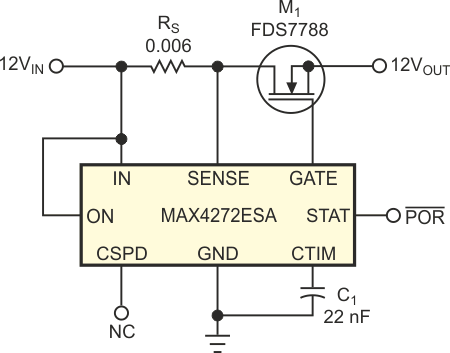Figure 1. A typical hot-swap controller circuit exhibits a 30-µsec short-circuit current pulse of 400 A peak.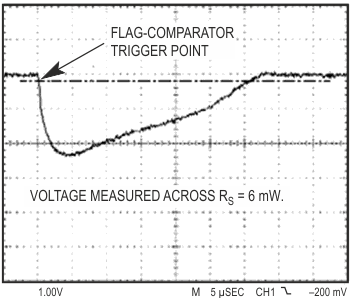Figure 2. The short-circuit current in Figure 1 is 400 A, decreasing to 100 A in 28 µsec.

You can limit the short-circuit current duration to less than 0.5 µsec by adding a Darlington pnp transistor, Q1, to speed the gate discharge (Figure 3). D1 allows the gate to charge normally at turn-on, but, at turn-off, the controller's 3-mA gate-discharge current is directed to the base of Q1. Q1 then acts quickly to discharge the gate, in less than 100 nsec. Thus, the high-current portion of the short circuit is limited to slightly more than the fast comparator's delay time of 350 nsec. The apparent reverse overshoot current and the steep rise in the waveform of Figure 4 arise from parasitic series inductance in the sense-resistor chip. The circuit of Figure 5 can limit short-circuit current to approximately 100 A for less than 200 nsec. The pnp transistor, Q1A, which triggers when the voltage across RS reaches approximately 600 mV, drives the npn transistor, Q1B, to quickly discharge M1’s gate capacitance. The steep voltage waveform aids quick triggering of the pnp transistor.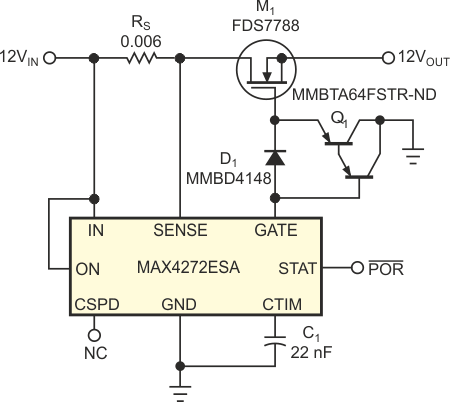Figure 3. The addition of Q1 increases the gate-pulldown current, limiting the short-circuit-current duration to less than 0.5 µsec.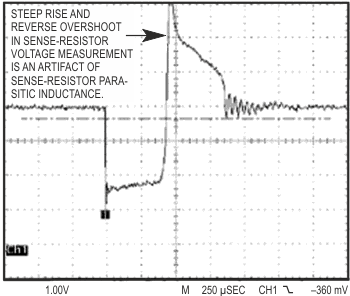Figure 4. The steep rise and reverse overshoot in Figure 3’s circuit are artifacts of sense-resistor parasitic inductance.

The oscilloscope's ground lead introduces an artifact, which appears as the leading-edge oscillation in Figure 6. Again, as in Figure 4, the apparent reverse-overshoot current and the steep rise in the waveform of Figure 6 arise from parasitic series inductance in the sense-resistor chip. C2 connects between the gate and source of M1 to reduce the positive-transient step voltage applied to the gate during a short circuit. Zener diode D1 reduces ID(ON) by limiting VGS to less than the 7 V available from the MAX4272. Although D1 is rated at 5.1 V when biased at 5 mA, it limits VGS to approximately 3.4 V in this circuit because only 100 µA of gate-charging current (zener-bias current) is available from the IC. The limited VGS lowers ID(ON) – at some expense to on-resistance – and allows a quicker turn-off of M1. You could also use D1 and C2 to some advantage in Figure 1 and Figure 3, to reduce ID(ON) during short circuits.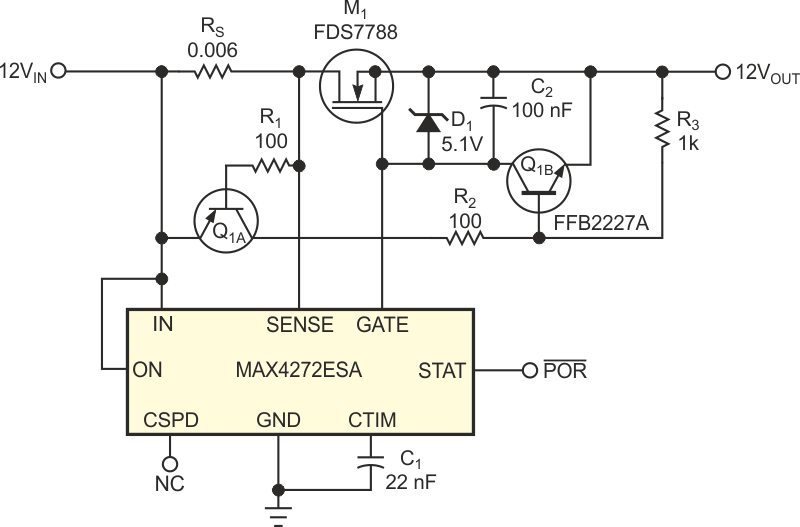Figure 5. This hot-swap controller has fast limiting of short-circuit current peaks.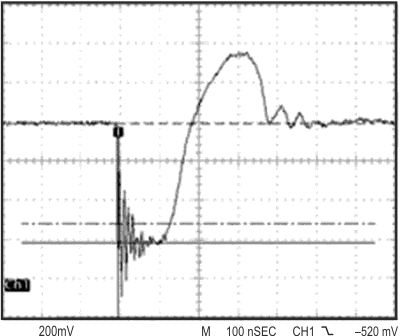Figure 6. This waveform depicts the short-circuit-current peaks for the circuit in Figure 4.

Either of the two circuits can protect a backplane power source by minimizing the energy dissipated when a hot-swap-controller circuit incurs a short circuit. The simpler circuit (Figure 3) dramatically shortens the short-circuit-current interval to somewhat less than 500 nsec, and the slightly more complex circuit (Figure 5) reduces the peak short-circuit current to 100 A, as well as truncating the pulse width to less than 200 nsec. You can apply either technique to most hot-swap-controller circuits. Individual results vary according to the impedance of the power source, the impedance of the short circuit, and the quality and attack time of the short circuit itself. Note that it is inordinately difficult to achieve a repeatable low-resistance short circuit by manual manipulation of a shorting bar. You require careful layout and low-ESR capacitors to create a power source with very low ESR.

EDN